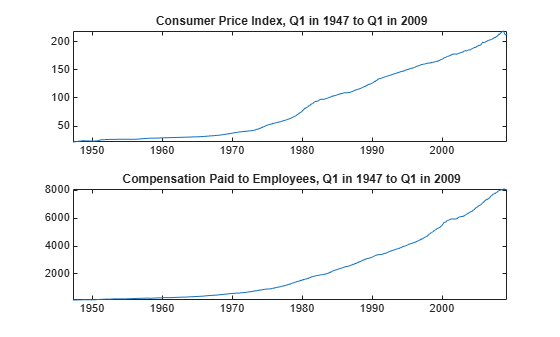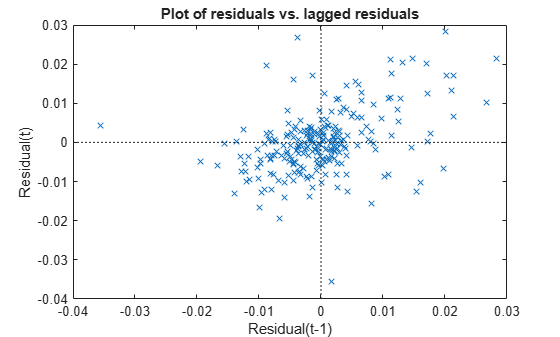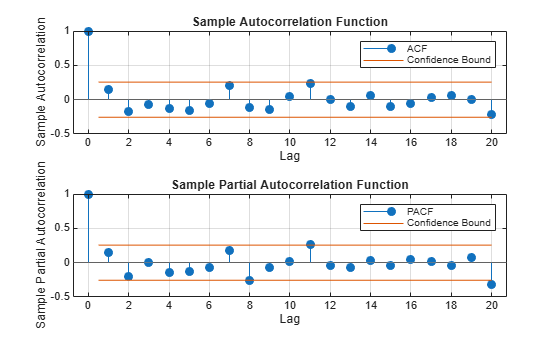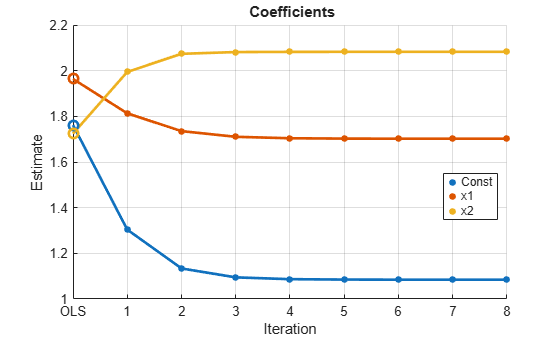# fgls

Feasible generalized least squares

## Syntax

coeff = fgls(X,y)
coeff = fgls(Tbl)
coeff = fgls(___,Name,Value)
[coeff,se,EstCoeffCov] = fgls(___)
coeff = fgls(ax,___)
[coeff,se,EstCoeffCov,iterPlots] = fgls(___)

## Description

example

coeff = fgls(X,y) returns coefficient estimates of the multiple linear regression model y = Xβ + ε using feasible generalized least squares (FGLS) by first estimating the covariance of the innovations process ε.NaNs in the data indicate missing values, which fgls removes using list-wise deletion. fgls sets Data = [X y], then it removes any row in Data containing at least one NaN. List-wise deletion reduces the effective sample size and changes the time base of the series.

example

coeff = fgls(Tbl) returns FGLS coefficient estimates using the predictor data in the first numPreds columns of the table Tbl and the response data in the last column.fgls removes all missing values in Tbl, indicated by NaNs, using list-wise deletion. In other words, fgls removes all rows in Tbl containing at least one NaN. List-wise deletion reduces the effective sample size and changes the time base of the series.

example

coeff = fgls(___,Name,Value) specifies options using one or more name-value pair arguments in addition to the input arguments in previous syntaxes. For example, you can choose the innovations covariance model, specify the number of iterations, and plot estimates after each iteration.

example

[coeff,se,EstCoeffCov] = fgls(___) additionally returns a vector of FGLS coefficient standard errors, se = sqrt(diag(EstCov)), and the FGLS estimated coefficient covariance matrix (EstCoeffCov).
coeff = fgls(ax,___) plots on the axes specified in ax instead of the axes of new figures. ax can precede any of the input argument combinations in the previous syntaxes.
[coeff,se,EstCoeffCov,iterPlots] = fgls(___) returns handles to plotted graphics objects. Use elements of iterPlots to modify properties of the plots after you create them.

## Examples

collapse all

Suppose the sensitivity of the U.S. Consumer Price Index (CPI) to changes in the paid compensation of employees (COE) is of interest.

Load the US macroeconomic data set. Plot the CPI and COE series.

load Data_USEconModel figure; subplot(2,1,1) plot(dates,DataTable.CPIAUCSL); title '{\bf Consumer Price Index, Q1 in 1947 to Q1 in 2009}'; datetick; axis tight; subplot(2,1,2); plot(dates,DataTable.COE); title '{\bf Compensation Paid to Employees, Q1 in 1947 to Q1 in 2009}'; datetick; axis tight;The series are nonstationary. Stabilize them by applying the log, and then the first difference.

CPI = diff(log(DataTable.CPIAUCSL)); COE = diff(log(DataTable.COE));

Regress CPI onto COE including an intercept to obtain ordinary least squares (OLS) estimates. Generate a lagged residual plot.

Mdl = fitlm(COE,CPI); figure; plotResiduals(Mdl,'lagged')There is an upward trend in the residual plot, which suggests that the innovations comprise an autoregressive process. This violates one of the classical linear model assumptions. Consequently, hypothesis tests based on the regression coefficients are incorrect, even asymptotically.

Estimate the regression coefficients using FGLS. By default, fgls includes an intercept in the regression model and imposes an AR(1) model on the innovations. Optionally, display the OLS and FGLS estimates by specifying 'final' for the 'display' name-value pair argument.

coeff = fgls(CPI,COE,'display','final');
OLS Estimates: | Coeff SE ------------------------ Const | 0.0122 0.0009 x1 | 0.4915 0.0686 FGLS Estimates: | Coeff SE ------------------------ Const | 0.0148 0.0012 x1 | 0.1961 0.0685 

If the COE series is exogenous with respect to the CPI, then the FGLS estimates (coeff) are consistent and asymptotically more efficient than the OLS estimates.

Suppose the sensitivity of the U.S. Consumer Price Index (CPI) to changes in the paid compensation of employees (COE) is of interest. This example enhances the analysis outlined in the example Estimate FGLS Coefficients Using Default Options.

Load the U.S. macroeconomic data set.

load Data_USEconModel

The series are nonstationary. Stabilize them by applying the log, and then the first difference.

CPI = diff(log(DataTable.CPIAUCSL)); COE = diff(log(DataTable.COE));

Regress CPI onto COE including an intercept to obtain OLS estimates. Plot correlograms for the residuals.

Mdl = fitlm(COE,CPI); u = Mdl.Residuals.Raw; figure; subplot(2,1,1) autocorr(u); subplot(2,1,2); parcorr(u);The correlograms suggest that the innovations have significant AR effects. According to Box-Jenkins Methodology, the innovations seem to comprise an AR(3) series.

Estimate the regression coefficients using FGLS. By default, fgls assumes that the innovations are autoregressive. Specify that the innovations are AR(3) using the 'arLags' name-value pair argument.

[coeff,se] = fgls(CPI,COE,'arLags',3,'display','final');
OLS Estimates: | Coeff SE ------------------------ Const | 0.0122 0.0009 x1 | 0.4915 0.0686 FGLS Estimates: | Coeff SE ------------------------ Const | 0.0148 0.0012 x1 | 0.1972 0.0684 

If the COE series is exogenous with respect to the CPI, then the FGLS estimates (coeff) are consistent and asymptotically more efficient than the OLS estimates.

Model the nominal GNP (GNPN) growth rate accounting for the effects of the growth rates of the consumer price index (CPI), real wages (WR), and the money stock (MS). Account for classical linear model departures.

Load the Nelson Plosser data set.

load Data_NelsonPlosser varIdx = [8,10,11,2]; % Variable indices idx = ~any(ismissing(DataTable),2); % Identify nonmissing values Tbl = DataTable(idx,varIdx); % Tabular array of variables T = sum(idx); % Sample size

Plot the series.

figure; for j = 1:4; subplot(2,2,j); plot(dates(idx),Tbl{:,j}); title(Tbl.Properties.VariableNames{j}); axis tight; end;All series appear nonstationary.

Apply the log, and then the first difference to each series.

dLogTbl = array2table(diff(log(Tbl{:,:})),... 'VariableNames',strcat(Tbl.Properties.VariableNames,'Rate'));

Regress GNPNRate onto the other variables in dLogTbl. Examine a scatter plot and correlograms of the residuals.

Mdl = fitlm(dLogTbl); figure; plotResiduals(Mdl,'caseorder'); axis tight;figure; subplot(2,1,1); autocorr(Mdl.Residuals.Raw); subplot(2,1,2); parcorr(Mdl.Residuals.Raw);The residuals appear to flare in, and so they exhibit heteroscedasticity. The correlograms suggest that there is no autocorrelation.

Estimate FGLS coefficients by accounting for the heteroscedasticity of the residuals. Specify that the estimated innovation covariance is diagonal with the squared residuals as weights.

fgls(dLogTbl,'innovMdl','HC0','display','final');
OLS Estimates: | Coeff SE --------------------------- Const | -0.0076 0.0085 CPIRate | 0.9037 0.1544 WRRate | 0.9036 0.1906 MSRate | 0.4285 0.1379 FGLS Estimates: | Coeff SE --------------------------- Const | -0.0102 0.0017 CPIRate | 0.8853 0.0169 WRRate | 0.8897 0.0294 MSRate | 0.4874 0.0291 

Create this regression model with ARMA(1,2) errors, where ${\epsilon }_{t}$ is Gaussian with mean 0 and variance 1.

$\begin{array}{c}{y}_{t}=1+{x}_{t}\left[\begin{array}{c}2\\ 3\end{array}\right]+{u}_{t}\\ {u}_{t}=0.6{u}_{t-1}+{\epsilon }_{t}-0.3{\epsilon }_{t-1}+0.1{\epsilon }_{t-1}.\end{array}$

beta = [2 3]; phi = 0.2; theta = [-0.3 0.1]; Mdl = regARIMA('AR',phi,'MA',theta,'Intercept',1,'Beta',beta,'Variance',1);

Mdl is a regARIMA model. You can access its properties using dot notation.

Simulate 500 periods of 2-D standard Gaussian values for ${x}_{t}$, and then simulate responses using Mdl.

numObs = 500; rng(1); % For reproducibility X = randn(numObs,2); y = simulate(Mdl,numObs,'X',X);

fgls supports AR(p) innovation models. You can convert an ARMA model polynomial to an infinite-lag AR model polynomial using arma2ar. By default, arma2ar returns the coefficients for the first 10 terms. After the conversion, determine how many lags of the resulting AR model are practically significant by checking the length of the returned vector of coefficients. Choose the number of terms that exceed 0.00001.

format long arParams = arma2ar(phi,theta)
arParams = 1×3 -0.100000000000000 0.070000000000000 0.031000000000000 
arLags = sum(abs(arParams) > 0.00001); format short

Some of the parameters have small magnitude. You might want to reduce the number of lags to include in the innovations model for fgls.

Estimate the coefficients and their standard errors using FGLS and the simulated data. Specify that the innovations comprise an AR(arLags) process.

[coeff,~,EstCoeffCov] = fgls(X,y,'innovMdl','AR','arLags',arLags)
coeff = 3×1 1.0372 2.0366 2.9918 
EstCoeffCov = 3×3 0.0026 -0.0000 0.0001 -0.0000 0.0022 0.0000 0.0001 0.0000 0.0024 

The estimated coefficients are close to their true values.

This example expands on the analysis in Estimate FGLS Coefficients of Models Containing ARMA Errors. Create this regression model with ARMA(1,2) errors, where ${\epsilon }_{t}$ is Gaussian with mean 0 and variance 1.

$\begin{array}{c}{y}_{t}=1+{x}_{t}\left[\begin{array}{c}2\\ 3\end{array}\right]+{u}_{t}\\ {u}_{t}=0.6{u}_{t-1}+{\epsilon }_{t}-0.3{\epsilon }_{t-1}+0.1{\epsilon }_{t-1}.\end{array}$

beta = [2 3]; phi = 0.2; theta = [-0.3 0.1]; Mdl = regARIMA('AR',phi,'MA',theta,'Intercept',1,'Beta',beta,'Variance',1);

Simulate 500 periods of 2-D standard Gaussian values for ${x}_{t}$, and then simulate responses using Mdl.

numObs = 500; rng(1); % For reproducibility X = randn(numObs,2); y = simulate(Mdl,numObs,'X',X);

Convert the ARMA model polynomial to an infinite-lag AR model polynomial using arma2ar. By default, arma2ar returns the coefficients for the first 10 terms. Find the number of terms that exceed 0.00001.

arParams = arma2ar(phi,theta); arLags = sum(abs(arParams) > 0.00001);

Estimate the regression coefficients using three iterations of FGLS, and specify the number of lags in the AR innovation model (arLags). Also, specify to plot the coefficient estimates and their standard errors for each iteration, and to display the final estimates and the OLS estimates in tabular form.

[coeff,~,EstCoeffCov] = fgls(X,y,'innovMdl','AR','arLags',arLags,... 'numIter',3,'plot',{'coeff','se'},'display','final');
OLS Estimates: | Coeff SE ------------------------ Const | 1.0375 0.0480 x1 | 2.0409 0.0473 x2 | 2.9860 0.0488 FGLS Estimates: | Coeff SE ------------------------ Const | 1.0372 0.0514 x1 | 2.0366 0.0470 x2 | 2.9919 0.0486The algorithm seems to converge after the first iteration, and the estimates are close to the OLS estimates, with the standard errors being slightly smaller.

Properties of iterative FGLS estimates in finite samples are difficult to establish. For asymptotic properties, one iteration of FGLS is sufficient. fgls supports iterative FGLS for experimentation.

If the estimates or standard errors show instability after successive iterations, then the estimated innovations covariance might be ill conditioned. Consider scaling the residuals using the 'resCond' name-value pair argument to improve the conditioning of the estimated innovations covariance.

## Input Arguments

collapse all

Predictor data for the multiple linear regression model, specified as a numObs-by-numPreds numeric matrix.

numObs is the number of observations and numPreds is the number of predictor variables.

Data Types: double

Response data for the multiple linear regression model, specified as a numObs-by-1 vector with numeric or logical entries.

Data Types: double | logical

Predictor and response data for the multiple linear regression model, specified as a numObs-by-numPreds + 1 tabular array.

The first numPreds variables of Tbl are the predictor data, and the last variable is the response data.

The predictor data must be numeric, and the response data must be numeric or logical.

Data Types: table

Axes on which to plot, specified as a vector of Axes objects with length equal to the number of plots specified by the plot name-value pair argument.

By default, fgls creates a separate figure for each plot.

Note

NaNs in X, y, or Tbl indicate missing values, and fgls removes observations containing at least one NaN. That is, to remove NaNs in X or y, the software merges them ([X y]), and then uses list-wise deletion to remove any row that contains at least one NaN. The software also removes any row of Tbl containing at least one NaN. Removing NaNs in the data reduces the sample size, and can also create irregular time series.

### Name-Value Pair Arguments

Specify optional comma-separated pairs of Name,Value arguments. Name is the argument name and Value is the corresponding value. Name must appear inside quotes. You can specify several name and value pair arguments in any order as Name1,Value1,...,NameN,ValueN.

Example: 'innovMdl','HC0','numIter',10,'plot','coeff' specifies White’s robust innovations covariance model, 10 iterations of FGLS, and to plot coefficient estimates after each iteration.

Variable names used in displays and plots of the results, specified as the comma-separated pair consisting of 'varNames' and a length numCoeffs cell vector of character vectors. The software truncates all variable names to the first five characters.

varNames must include variable names for all variables in the model, such as an intercept term (e.g., 'Const') or higher-order terms (e.g., 'x1^2' or 'x1:x2'). If the value of 'intercept' is true, then the first element is the name of the intercept. The order of all other elements corresponds to the order of the columns of X or predictor variables in Tbl.

If 'Intercept' is true, then its default name is 'Const'. The default variable names for:

• The predictor variables in X is the cell vector of character vectors {'x1','x2',...}

• The tabular array Tbl is the property Tbl.Properties.VariableNames

Example: 'varNames',{'Const','AGE','BBD'}

Data Types: cell | string

Indicate whether to include model intercept when fgls fits the model, specified as the comma-separated pair consisting of 'intercept' and true or false. The number of model coefficients, numCoeffs, is numPreds + intercept.

ValueDescription
trueInclude an intercept in the model.
falseExclude an intercept from the model.

Example: 'intercept',false

Data Types: logical

Model for the innovations covariance estimate, specified as the comma-separated pair consisting of 'innovMdl' and a character vector.

Set 'innovMdl' to specify the structure of the innovations covariance estimator $\stackrel{^}{\Omega }$.

• For diagonal innovations covariance models (i.e., models with heteroscedasticity), $\stackrel{^}{\Omega }=diag\left(\omega \right),$ where ω = {ωi; i = 1,...,T} is a vector of innovation variance estimates for the observations, and T = numObs.

fgls estimates the data-driven vector ω using the corresponding model residuals (ε), their leverages ${h}_{i}={x}_{i}{\left({X}^{\prime }X\right)}^{-1}{x}_{i}^{\prime },$ and the degrees of freedom dfe.

Use this table to choose 'innovMdl'.

ValueWeightReference
'CLM'

${\omega }_{i}=\frac{1}{dfe}\sum _{i=1}^{T}{\epsilon }_{i}^{2}$


'HC0'

${\omega }_{i}={\epsilon }_{i}^{2}$


'HC1'

${\omega }_{i}=\frac{T}{dfe}{\epsilon }_{i}^{2}$


'HC2'

${\omega }_{i}=\frac{{\epsilon }_{i}^{2}}{1-{h}_{i}}$


'HC3'

${\omega }_{i}=\frac{{\epsilon }_{i}^{2}}{{\left(1-{h}_{i}\right)}^{2}}$


'HC4'

${\omega }_{i}=\frac{{\epsilon }_{i}^{2}}{{\left(1-{h}_{i}\right)}^{{d}_{i}}}$

where ${d}_{i}=\mathrm{min}\left(4,\frac{{h}_{i}}{\overline{h}}\right),$



• For full innovation covariance models (i.e., models having heteroscedasticity and autocorrelation), specify 'AR'. The software imposes an AR(p) model on the innovations, and constructs $\stackrel{^}{\Omega }$ using the number of lags, p, specified by the name-value pair argument arLags and the Yule-Walker equations.

If numIter is 1 and you specify InnovCov0, then fgls ignores InnovMdl.

Example: 'innovMdl',HC0

Data Types: char | string

Number of lags to include in the AR innovations model, specified as the comma-separated pair consisting of 'arLags' and a positive integer.

If innovMdl is not 'AR' (i.e., for diagonal models), then the software ignores the value of 'arLags'.

For general ARMA innovations models, convert to the equivalent AR form by:

• Constructing the ARMA innovations model lag operator polynomial using LagOp. Then, divide the AR polynomial by the MA polynomial using, e.g., mrdivide. The result is the infinite-order, AR representation of the ARMA model.

• Using arma2ar, which returns the coefficients of the infinite-order, AR representation of the ARMA model.

Example: 'arLags',4

Data Types: double

Initial innovations covariance, specified as the comma-specified pair consisting of 'InnovCov0' and a vector of positive scalars, positive semidefinite matrix, or a positive definite matrix.

InnovCov0 replaces the data-driven estimate of the innovations covariance ($\stackrel{^}{\Omega }$) in the first iteration of GLS.

• For diagonal innovations covariance models (i.e., models with heteroscedasticity), specify a numObs-by-1 vector. InnovCov0(j) is the variance of innovation j.

• For full innovation covariance models (i.e., models having heteroscedasticity and autocorrelation), specify a numObs-by-numObs matrix. InnovCov0(j,k) is the covariance of innovations j and k.

• By default, fgls uses a data-driven $\stackrel{^}{\Omega }$ (see innovMdl).

Data Types: double

Number of iterations to implement for the FGLS algorithm, specified as the comma-separated pair consisting of 'numIter' and a positive integer.

fgls estimates the innovations covariance ($\stackrel{^}{\Omega }$) at each iteration from the residual series according to the innovations covariance model (innovMdl). Then, the software computes the GLS estimates of the model coefficients.

Example: 'numIter',10

Data Types: double

Flag indicating to scale the residuals at each iteration of FGLS, specified as the comma-separated pair consisting of 'resCond' and true or false.

ValueDescription
truefgls scales the residuals at each iteration.
falsefgls does not scale the residuals at each iteration.

Scaling the residuals at each iteration of FGLS tends to improve the conditioning of the estimation of the innovations covariance ($\stackrel{^}{\Omega }$).

Data Types: logical

Command Window display control, specified as the comma-separated pair consisting of 'display' and value in this table.

ValueDescription
'final'Display the final estimates.
'iter'Display the estimates after each iteration.
'off'Suppress Command Window display.

fgls shows estimation results in tabular form.

Example: 'display','iter'

Data Types: char | string

Control for plotting results after each iteration, specified as the comma-separated pair consisting of 'plot' and a character vector or cell array of character vectors.

To examine the convergence of the FGLS algorithm, it is good practice to specify plotting the estimates for each iteration. This table contains the available values.

ValueDescription
'allPlot the estimated coefficients, their standard errors, and the residual mean-squared error (MSE) on separate plots.
'coeff'Plot the estimated coefficients.
'mse'Plot the MSE.
'off'Do not plot the results.
'se'Plot the estimated coefficient.

Data Types: char | string

## Output Arguments

collapse all

FGLS coefficient estimates, returned as a numCoeffs-by-1 numeric vector.

The order of the estimates corresponds to the order of the predictor matrix columns or Tbl.VariableNames. For example, in a model with an intercept, the value of ${\stackrel{^}{\beta }}_{1}$ (corresponding to the predictor x1) is in position 2 of coeff.

Coefficient standard error estimates, returned as a numCoeffs-by-1 numeric. The elements of se are sqrt(diag(EstCoeffCov)).

The order of the estimates corresponds to the order of the coefficients in coeff. For example, in a model with an intercept, the estimated standard error of ${\stackrel{^}{\beta }}_{1}$ (corresponding to the predictor x1) is in position 2 of se, and is the square root of the value in position (2,2) of EstCoeffCov.

Coefficient covariance estimate, returned as a numCoeffs-by-numCoeffs numeric matrix.

The order of the rows and columns of EstCoeffCov corresponds to the order of the coefficients in coeff. For example, in a model with an intercept, the estimated covariance of ${\stackrel{^}{\beta }}_{1}$ (corresponding to the predictor x1) and ${\stackrel{^}{\beta }}_{2}$ (corresponding to the predictor x2) are in positions (2,3) and (3,2) of EstCoeffCov, respectively.

Handles to plotted graphics objects, returned as a structure array of graphics objects. iterPlots contains unique plot identifiers, which you can use to query or modify properties of the plot.

iterPlots is not available if the value of the plot name-value pair argument is 'off'.

collapse all

### Feasible Generalized Least Squares

Feasible generalized least squares (FGLS) estimates the coefficients of a multiple linear regression model and their covariance matrix in the presence of nonspherical innovations with an unknown covariance matrix.

Let yt = Xtβ + εt be a multiple linear regression model, where the innovations process εt is Gaussian with mean 0, but with true, nonspherical covariance matrix Ω (e.g., the innovations are heteroscedastic or autocorrelated). Also, suppose that the sample size is T and there are p predictors (including an intercept). Then, the FGLS estimator of β is

${\stackrel{^}{\beta }}_{FGLS}={\left({X}^{\top }{\stackrel{^}{\Omega }}^{-1}X\right)}^{-1}{X}^{\top }{\stackrel{^}{\Omega }}^{-1}y,$

where $\stackrel{^}{\Omega }$ is an innovations covariance estimate based on a model (e.g., innovations process forms an AR(1) model). The estimated coefficient covariance matrix is

${\stackrel{^}{\Sigma }}_{FGLS}={\stackrel{^}{\sigma }}_{FGLS}^{2}{\left({X}^{\top }{\stackrel{^}{\Omega }}^{-1}X\right)}^{-1},$

where

${\stackrel{^}{\sigma }}_{FGLS}^{2}={y}^{\top }\left[{\stackrel{^}{\Omega }}^{-1}-{\stackrel{^}{\Omega }}^{-1}X{\left({X}^{\top }{\stackrel{^}{\Omega }}^{-1}X\right)}^{-1}{X}^{\top }{\stackrel{^}{\Omega }}^{-1}\right]y/\left(T-p\right).$

FGLS estimates are computed as follows:

1. OLS is applied to the data, and then residuals $\left({\stackrel{^}{\epsilon }}_{t}\right)$ are computed.

2. $\stackrel{^}{\Omega }$ is estimated based on a model for the innovations covariance.

3. ${\stackrel{^}{\beta }}_{FGLS}$ is estimated, along with its covariance matrix ${\stackrel{^}{\Sigma }}_{FGLS}.$

4. Optional: This process can be iterated by performing the following steps until ${\stackrel{^}{\beta }}_{FGLS}$ converges.

1. Compute the residuals of the fitted model using the FGLS estimates.

2. Apply steps 2–3.

If $\stackrel{^}{\Omega }$ is a consistent estimator of $\Omega$ and the predictors that comprise X are exogenous, then FGLS estimators are consistent and efficient.

Asymptotic distributions of FGLS estimators are unchanged by repeated iteration. However, iterations might change finite sample distributions.

### Generalized Least Squares

Generalized least squares (GLS) estimates the coefficients of a multiple linear regression model and their covariance matrix in the presence of nonspherical innovations with known covariance matrix.

The setup and process for obtaining GLS estimates is the same as in FGLS, but replace $\stackrel{^}{\Omega }$ with the known innovations covariance matrix $\Omega$.

In the presence of nonspherical innovations, and with known innovations covariance, GLS estimators are unbiased, efficient, and consistent, and hypothesis tests based on the estimates are valid.

### Weighted Least Squares

Weighted least squares (WLS) estimates the coefficients of a multiple linear regression model and their covariance matrix in the presence of uncorrelated, but heteroscedastic innovations with known, diagonal covariance matrix.

The setup and process to obtain WLS estimates is the same as in FGLS, but replace $\stackrel{^}{\Omega }$ with the known, diagonal matrix of weights, typically the diagonal elements are the inverse of the variances of the innovations.

In the presence of heteroscedastic innovations, and when the variances of the innovations are known, WLS estimators are unbiased, efficient, and consistent, and hypothesis tests based on the estimates are valid.

## Tips

• To obtain standard generalized least squares (GLS) estimates:

• To obtain WLS estimates, set the InnovCov0 name-value pair argument to a vector of inverse weights (e.g., innovations variance estimates).

• In specific models and with repeated iterations, scale differences in the residuals might produce a badly conditioned estimated innovations covariance and induce numerical instability. If you set 'resCond',true, then conditioning improves.

## Algorithms

• In the presence of nonspherical innovations, GLS produces efficient estimates relative to OLS, and consistent coefficient covariances, conditional on the innovations covariance. The degree to which fgls maintains these properties depends on the accuracy of both the model and estimation of the innovations covariance.

• Rather than estimate FGLS estimates the usual way, fgls uses methods that are faster and more stable, and are applicable to rank-deficient cases.

• Traditional FGLS methods, such as the Cochrane-Orcutt procedure, use low-order, autoregressive models. These methods, however, estimate parameters in the innovations covariance matrix using OLS, where fgls uses maximum likelihood estimation (MLE) .

 Cribari-Neto, F. "Asymptotic Inference Under Heteroskedasticity of Unknown Form." Computational Statistics & Data Analysis. Vol. 45, 2004, pp. 215–233.

 Hamilton, J. D. Time Series Analysis. Princeton, NJ: Princeton University Press, 1994.

 Judge, G. G., W. E. Griffiths, R. C. Hill, H. Lutkepohl, and T. C. Lee. The Theory and Practice of Econometrics. New York, NY: John Wiley & Sons, Inc., 1985.

 Kutner, M. H., C. J. Nachtsheim, J. Neter, and W. Li. Applied Linear Statistical Models. 5th ed. New York: McGraw-Hill/Irwin, 2005.

 MacKinnon, J. G., and H. White. "Some Heteroskedasticity-Consistent Covariance Matrix Estimators with Improved Finite Sample Properties." Journal of Econometrics. Vol. 29, 1985, pp. 305–325.

 White, H. "A Heteroskedasticity-Consistent Covariance Matrix and a Direct Test for Heteroskedasticity." Econometrica. Vol. 48, 1980, pp. 817–838.